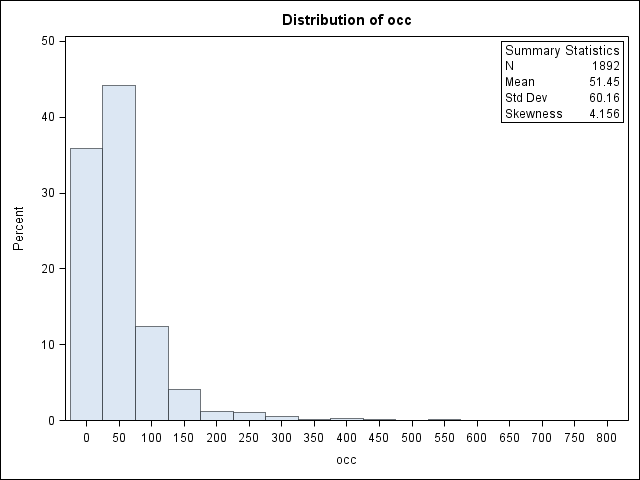## Distribution plot from proc univariate

Can I please ask what do this errors mean? How can I resolve it? Thank you very much.```560  proc univariate data=temp;
561      var occ;
562      histogram / midpoints=0 to 600 by 50
563      lognormal
564     weibull
565      gamma;
566      inset n mean(5.3) std='Std Dev'(5.3) skewness(5.3)
567      / pos = ne  header = 'Summary Statistics';
578  run;

NOTE: Since a threshold parameter (THETA) was not specified for the lognormal fit for occ, a
zero threshold is assumed.
ERROR: The smallest value of occ is less than or equal to the threshold parameter (THETA) for
the lognormal fit.
NOTE: The lognormal threshold parameter (THETA) must be less than 0.
NOTE: The lognormal curve was not drawn because estimates could not be computed for all
parameters.
NOTE: Since a threshold parameter (THETA) was not specified for the Weibull fit for occ, a
zero threshold is assumed.
ERROR: The smallest value of occ is less than or equal to the threshold parameter (THETA) for
the Weibull fit.
NOTE: The Weibull threshold parameter (THETA) must be less than 0.
NOTE: The Weibull curve was not drawn because estimates could not be computed for all
parameters.
NOTE: Since a threshold parameter (THETA) was not specified for the gamma fit for occ, a zero
threshold is assumed.
ERROR: The smallest value of occ is less than or equal to the threshold parameter (THETA) for
the gamma fit.
NOTE: The gamma threshold parameter (THETA) must be less than 0.
NOTE: The gamma curve was not drawn because estimates could not be computed for all parameters.
NOTE: At least one W.D format was too small for the number to be printed. The decimal may be
shifted by the "BEST" format.
NOTE: PROCEDURE UNIVARIATE used (Total process time):
real time           0.19 seconds
cpu time            0.04 seconds```

## Re: Distribution plot from proc univariate

In three=parameter families that have a threshold parameters, the data must be strictly larger than the values of the threshold parameter.  The reason is that the distributions you are fitting have a term that looks like

log( x[i] - theta )

or

(x[i] - theta)**c.

The LOG function is undefined when its argument is zero or negative. Similarly, the "raise to power function" is undefined for negative arguments when  c is not an integer. (For example, c=1/2 is the square roo function.)

The most likely reason for these errors is that you have data values that are zero. Your choices include:

1. Model the data by using different distributions
2. Specify a value for the threshold parameters that is strictly less than the smallest data value
3. Delete the bad data values if they are coding errors
Discussion stats